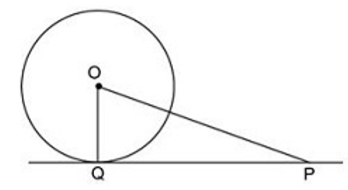# SSC CHSL 13 March 2018 Morning Shift

Instructions

For the following questions answer them individually

Question 1

7/15 of 5/3 of a number is greater than 7/9 of 3/5 of the same number by 9. Find the quadruple of the number.

Question 2

In a farm, in addition to 48 hens there are 42 sheep and 11 oxen with some shepherds. If the total number of feet be 224 more than the number of heads, find the number of shepherd.

Question 3

Which of the following value of 'X' satisfies the equation $$81^{4x+4}=243^{2x+8}$$ ?

Question 4

What will be the value of $$r^{2}+p^{2}+s^{2}-rp-ps-rs$$ where r = 1, p = 2, s = 3

Question 5

Calculate the length (in cm) of PQ. if the radius of the circle is 7 cm and the line through the centre 0 meets the tangent at point P such that OP = 25 cm.Question 6

Consider the following figure shown below and choose which of the following equation is correct about the similarity of both triangles?

Question 7

If y% of y is 289, then what is the value of y?

Question 8

P, Q and R are partners in a company. In any year P receives 1/9 part of profit, Q receives 1/6 part of profit and R receives Rs 130000. How much amount (in Rs) will P get as profit?

Question 9

In a container, two types of liquids P and Q are in the ratio of 3 : 7. If 10 litres mixture is drawn off from the container and filled with 6 litres of liquid Q, then the ratio of P and Q becomes 1 : 3. What was the original quantity (in litres) of mixture?

Question 10

The average weight of 35 apples is 50 grams. If some apples of weight 40 grams each were removed, then average weight becomes 57.5 grams. How many apples of weight 40 grams were removed?

OR